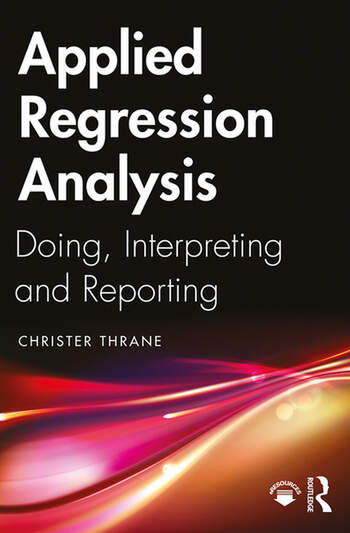# Applied Regression Analysis: Doing, Interpreting and Reporting

## 1st Edition

Christer Thrane

Routledge
Published October 28, 2019
Textbook - 192 Pages - 65 B/W Illustrations
ISBN 9781138335486 - CAT# K393619

For Instructors Request Inspection Copy

was \$60.95

USD\$48.76

SAVE ~\$12.19

FREE Standard Shipping!

## Preview

### Summary

This book is an introduction to regression analysis, focusing on the practicalities of doing regression analysis on real-life data.

Contrary to other textbooks on regression, this book is based on the idea that you do not necessarily need to know much about statistics and mathematics to get a firm grip on regression and perform it to perfection. This non-technical point of departure is complemented by practical examples of real-life data analysis using statistics software such as Stata, R and SPSS. Parts 1 and 2 of the book cover the basics, such as simple linear regression, multiple linear regression, how to interpret the output from statistics programs, significance testing and the key regression assumptions. Part 3 deals with how to practically handle violations of the classical linear regression assumptions, regression modeling for categorical y-variables and instrumental variable (IV) regression. Part 4 puts the various purposes of, or motivations for, regression into the wider context of writing a scholarly report and points to some extensions to related statistical techniques.

This book is written primarily for those who need to do regression analysis in practice, and not only to understand how this method works in theory. The book’s accessible approach is recommended for students from across the social sciences.

#### Instructors

We provide complimentary e-inspection copies of primary textbooks to instructors considering our books for course adoption.